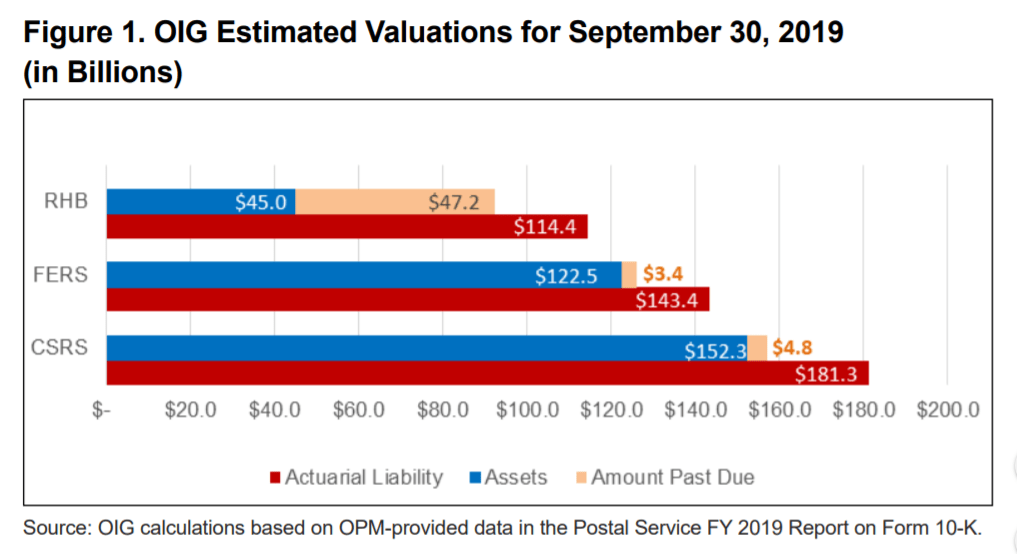# Bar graph: Combine one stacked bar with one dodged bar

I'm trying to recreate a bar graph found on page 4 of the following report:The figure has three bars with the first two stacked and the third dodged next to it. I've seen iterations of this question but none that recreate the figure in this exact way.

Here is the data:

``````a <- rep(c('RHB', 'FERS', 'CSRS'), 3)
b <- c(rep('Assets', 3), rep('Amount Past Due', 3),
rep('Actuarial Liability', 3))
c <- c(45.0, 122.5, 152.3, 47.2, 3.4, 4.8, 114.4, 143.4, 181.3)

df <- data.frame(a,b,c)
names(df) <- c('Fund', 'Condition', 'Value')
``````

And what I've managed so far:

``````p <- ggplot(subset_data, aes(fill=Condition, y=Value, x=Fund)) +
geom_bar(position="stack", stat="identity") +
coord_flip()
``````

I'm not partial to `ggplot` so if there's another tool that works better I'm ok using another package.